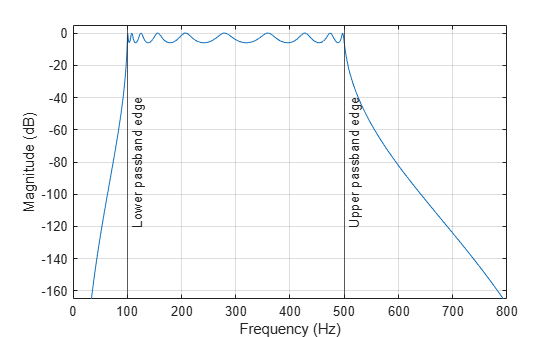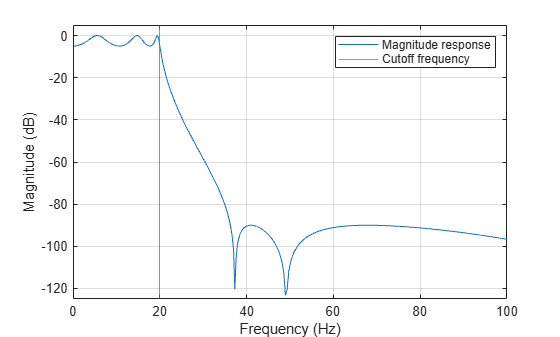# bilinear

Bilinear transformation method for analog-to-digital filter conversion

## Syntax

``[zd,pd,kd] = bilinear(z,p,k,fs)``
``[numd,dend] = bilinear(num,den,fs)``
``[Ad,Bd,Cd,Dd] = bilinear(A,B,C,D,fs)``
``[___] = bilinear(___,fp)``

## Description

example

````[zd,pd,kd] = bilinear(z,p,k,fs)` converts the s-domain transfer function in pole-zero form specified by `z`, `p`, `k` and sample rate `fs` to a discrete equivalent.```
````[numd,dend] = bilinear(num,den,fs)` converts the s-domain transfer function specified by numerator `num` and denominator `den` to a discrete equivalent.```

example

````[Ad,Bd,Cd,Dd] = bilinear(A,B,C,D,fs)` converts the continuous-time state-space system in matrices `A`, `B`, `C`, and `D` to a discrete-time system.```

example

````[___] = bilinear(___,fp)`uses parameter `fp` as "match" frequency to specify prewarping.```

## Examples

collapse all

Design the prototype for a 10th-order Chebyshev type I filter with 6 dB of ripple in the passband. Convert the prototype to state-space form.

```[z,p,k] = cheb1ap(10,6); [A,B,C,D] = zp2ss(z,p,k); ```

Transform the prototype to a bandpass filter such that the equivalent digital filter has a passband with edges at 100 Hz and 500 Hz when sampled at a rate ${\mathit{f}}_{\mathit{s}}=2\text{\hspace{0.17em}}\mathrm{kHz}$. For the transformation, specify prewarped band edges ${\mathit{u}}_{1}\text{\hspace{0.17em}}$and ${\mathit{u}}_{2}$ in rad/s, a center frequency ${\mathit{W}}_{\mathit{o}}=\sqrt{{\mathit{u}}_{1}{\mathit{u}}_{2}}$, and a bandwidth ${\mathit{B}}_{\mathit{w}}={\mathit{u}}_{2}-{\mathit{u}}_{1}$.

```fs = 2e3; f1 = 100; u1 = 2*fs*tan(f1*(2*pi/fs)/2); f2 = 500; u2 = 2*fs*tan(f2*(2*pi/fs)/2); [At,Bt,Ct,Dt] = lp2bp(A,B,C,D,sqrt(u1*u2),u2-u1);```

Compute the frequency response of the analog filter using `freqs`. Plot the magnitude response and the prewarped frequency band edges.

```[b,a] = ss2tf(At,Bt,Ct,Dt); [h,w] = freqs(b,a,2048); plot(w,mag2db(abs(h))) xline([u1 u2],"-",["Lower" "Upper"]+" passband edge", ... LabelVerticalAlignment="middle") ylim([-165 5]) xlabel("Angular frequency (rad/s)") ylabel("Magnitude (dB)") grid```Use the `bilinear` function to create a digital bandpass filter with sample rate ${\mathit{f}}_{\mathit{s}}$.

`[Ad,Bd,Cd,Dd] = bilinear(At,Bt,Ct,Dt,fs);`

Convert the digital filter from state-space form to second-order sections and compute the frequency response using `freqz`. Plot the magnitude response and the passband edges.

```[hd,fd] = freqz(ss2sos(Ad,Bd,Cd,Dd),2048,fs); plot(fd,mag2db(abs(hd))) xline([f1 f2],"-",["Lower" "Upper"]+" passband edge", ... LabelVerticalAlignment="middle") ylim([-165 5]) xlabel("Frequency (Hz)") ylabel("Magnitude (dB)") grid```Design a 6th-order elliptic analog lowpass filter with 5 dB of ripple in the passband, a stopband attenuation of 90 dB, and a cutoff frequency ${\mathit{f}}_{\mathit{c}}=20\text{\hspace{0.17em}}\mathrm{Hz}$.

```fc = 20; [z,p,k] = ellip(6,5,90,2*pi*fc,"s");```

Visualize the magnitude response of the analog elliptic filter. Display the cutoff frequency.

```[num,den] = zp2tf(z,p,k); [h,w] = freqs(num,den,1024); plot(w/(2*pi),mag2db(abs(h))) xline(fc,Color=[0.8500 0.3250 0.0980]) axis([0 100 -125 5]) grid legend(["Magnitude response" "Cutoff frequency"]) xlabel("Frequency (Hz)") ylabel("Magnitude (dB)")```Use the `bilinear` function to transform the analog filter to a discrete-time IIR filter. Specify a sample rate ${\mathit{f}}_{\mathit{s}}=200\text{\hspace{0.17em}}\mathrm{Hz}$ and a prewarping match frequency ${\mathit{f}}_{\mathit{p}}=20\text{\hspace{0.17em}}\mathrm{Hz}$.

```fs = 200; fp = 20; [zd,pd,kd] = bilinear(z,p,k,fs,fp);```

Visualize the magnitude response of the discrete-time filter. Display the cutoff frequency.

```[hd,fd] = freqz(zp2sos(zd,pd,kd),[],fs); plot(fd,mag2db(abs(hd))) xline(fc,Color=[0.8500 0.3250 0.0980]) axis([0 100 -125 5]) grid legend(["Magnitude response" "Cutoff frequency"]) xlabel("Frequency (Hz)") ylabel("Magnitude (dB)")```## Input Arguments

collapse all

Zeros, poles, and gain of the s-domain transfer function, specified as two column vectors and a scalar.

Sample rate, specified as a positive scalar.

Numerator and denominator coefficients of the analog transfer function, specified as row vectors.

State-space representation in the s-domain, specified as matrices. If the system has p inputs and q outputs and is described by n state variables, then `A` is n-by-n, `B` is n-by-p, `C` is q-by-n, and `D` is q-by-p.

Data Types: `single` | `double`

Match frequency, specified as a positive scalar.

## Output Arguments

collapse all

Zeros, poles, and gain of the z-domain transfer function, returned as column vectors and a scalar.

Numerator and denominator coefficients of the digital transfer function, returned as row vectors.

State-space representation in the z-domain, returned as matrices. If the system is described by n state variables and has q outputs, then `Ad` is n-by-n, `Bd` is n-by-1, `Cd` is q-by-n, and `Dd` is q-by-1.

Data Types: `single` | `double`

## Algorithms

collapse all

The bilinear transformation is a mathematical mapping of variables. In digital filtering, it is a standard method of mapping the s or analog plane into the z or digital plane. It transforms analog filters, designed using classical filter design techniques, into their discrete equivalents.

The bilinear transformation maps the s-plane into the z-plane by

`${H\left(z\right)=H\left(s\right)|}_{s=2{f}_{s}\frac{z-1}{z+1}}.$`

This transformation maps the jΩ axis (from Ω = –∞ to +∞) repeatedly around the unit circle (e, from ω = –π to π) by

`$\omega =2{\mathrm{tan}}^{-1}\left(\frac{\Omega }{2{f}_{s}}\right).$`

`bilinear` can accept an optional parameter `Fp` that specifies prewarping. `fp`, in hertz, indicates a "match" frequency for which the frequency responses before and after mapping match exactly. In prewarped mode, the bilinear transformation maps the s-plane into the z-plane with

`${H\left(z\right)=H\left(s\right)|}_{s=\frac{2\pi {f}_{p}}{\mathrm{tan}\left(\pi \frac{{f}_{p}}{{f}_{s}}\right)}\frac{z-1}{z+1}}.$`

With the prewarping option, `bilinear` maps the jΩ axis (from Ω = –∞ to +∞) repeatedly around the unit circle (e, from ω = –π to π) by

`$\omega =2{\mathrm{tan}}^{-1}\left(\frac{\Omega \mathrm{tan}\left(\pi \frac{{f}_{p}}{{f}_{s}}\right)}{2\pi {f}_{p}}\right).$`

In prewarped mode, `bilinear` matches the frequency 2πfp (in radians per second) in the s-plane to the normalized frequency 2πfp/fs (in radians per second) in the z-plane.

The `bilinear` function works with three different linear system representations: zero-pole-gain, transfer function, and state-space form.

`bilinear` uses one of two algorithms depending on the format of the input linear system you supply. One algorithm works on the zero-pole-gain format and the other on the state-space format. For transfer function representations, `bilinear` converts to state-space form, performs the transformation, and converts the resulting state-space system back to transfer function form.

### Zero-Pole-Gain Algorithm

For a system in zero-pole-gain form, `bilinear` performs four steps:

1. If `fp` is present, it prewarps:

```fp = 2*pi*fp; fs = fp/tan(fp/fs/2) ```

otherwise, `fs = 2*fs`.

2. It strips any zeros at ±∞ using

```z = z(finite(z)); ```
3. It transforms the zeros, poles, and gain using

```pd = (1+p/fs)./(1-p/fs); % Do bilinear transformation zd = (1+z/fs)./(1-z/fs); kd = real(k*prod(fs-z)./prod(fs-p)); ```
4. It adds extra zeros at -1 so the resulting system has equivalent numerator and denominator order.

### State-Space Algorithm

An analog system in state space form is given by

`$\begin{array}{l}\stackrel{˙}{x}=Ax+Bu\\ y=Cx+Du\end{array}$`

. This system is converted to the discrete form using state-space equations as follows:

To convert an analog system in state-space form, `bilinear` performs two steps:

1. If `fp` is present, let

`$\lambda =\frac{\pi {f}_{p}}{\mathrm{tan}\left(\pi {f}_{p}/{f}_{s}\right)}.$`

If `fp` is not present, let λ=fs.

2. Compute `Ad`, `Bd`, `Cd`, and `Dd` in terms of `A`, `B`, `C`, and `D` using

`$\begin{array}{l}{A}_{d}={\left(}^{I}\left(I+A\frac{1}{2\lambda }\right),\\ {B}_{d}=\frac{1}{\sqrt{\lambda }}{\left(}^{I}B,\\ {C}_{d}=\frac{1}{\sqrt{\lambda }}C{\left(}^{I},\\ {D}_{d}=\frac{1}{2\lambda }C{\left(}^{I}B+D.\end{array}$`

### Transfer Function

For a system in transfer function form, `bilinear` converts an s-domain transfer function given by `num` and `den` to a discrete equivalent. Row vectors `num` and `den` specify the coefficients of the numerator and denominator, respectively, in descending powers of s. Let B(s) be the numerator polynomial and A(s) be the denominator polynomial. The transfer function is:

`$\frac{B\left(s\right)}{A\left(s\right)}=\frac{B\left(1\right){s}^{n}+\cdots +B\left(n\right)s+B\left(n+1\right)}{A\left(1\right){s}^{m}+\cdots +A\left(m\right)s+A\left(m+1\right)}$`

`fs` is the sample rate in hertz. `bilinear` returns the discrete equivalent in row vectors `numd` and `dend` in descending powers of z (ascending powers of z–1). `fp` is the optional match frequency, in hertz, for prewarping.

 Oppenheim, Alan V., and Ronald W. Schafer, with John R. Buck. Discrete-Time Signal Processing. Upper Saddle River, NJ: Prentice Hall, 1999.

 Parks, Thomas W., and C. Sidney Burrus. Digital Filter Design. New York: John Wiley & Sons, 1987.